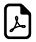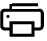Math Five Times Tables - Half Value Trick5 Times Multiplication Table Trick

An online five times table tricks.Easy way to learn the 5 times table.Five Times Table Simple Easy Learningcan write to
can write to
can write to
can write to
can write to
can write to
can write to
can write to
can write to
can write to
can write to

How 5 times table works?

When you multiply 5 with anything, you have to use the Half-Value 10 times table method. Here's an example: 2 x 5 is 10. Just write 10 times table, 2 x 10 = 20 , half value of 20 is 10. So answer for 2 x 5 = 10.

Trick to multiply an even number by 5

 2 x 5 = 10 4 x 5 = 20 6 x 5 = 30

If we multiply by an even number, your answer should end in 0.

Trick to multiply an odd number by 5

 3 x 5 = 15 5 x 5 = 25 7 x 5 = 35

If we multiply by an odd number, your answer should end in 5

Think 5 times.Think 10 times by 2 !!!

What math times time do you want to generate ?Times Table Tricks 2 to 11►

2 Times Table Trick

4 Times Table Trick

5 Times Table Trick

6 & 7 Times Table Trick

8 Times Table TrickTop Calculators ►

Tax Calculator, Calories Burned Calculator, PERT, SD Calculator, Dog Age Calculator, Children Equal Playing Time Calculator